# Determinants Class 12 NCERT Solutions

## In this pdf file you can see answers of following Questions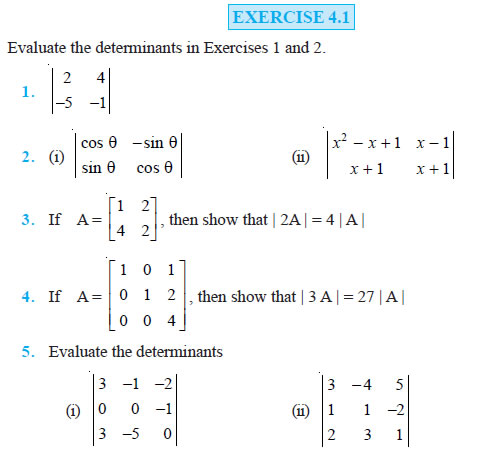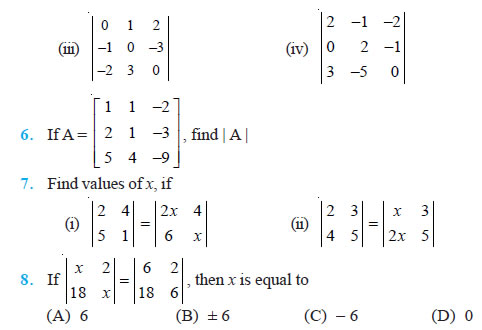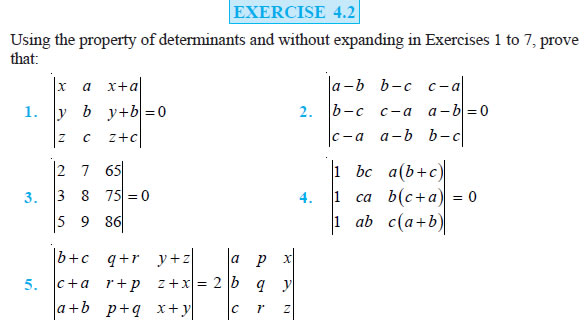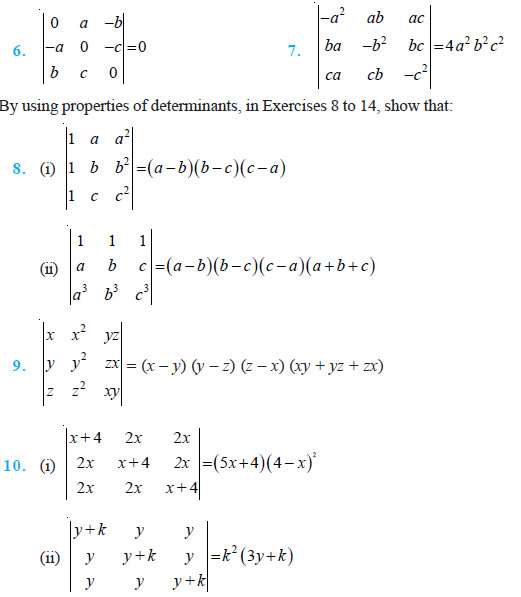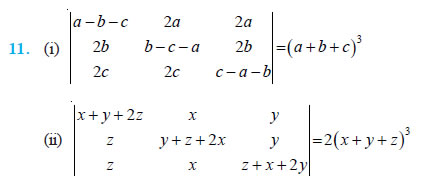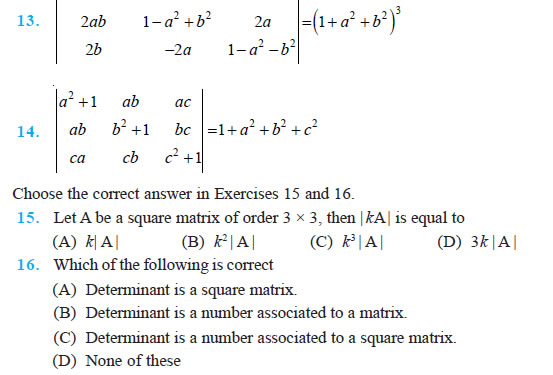### EXERCISE 4.3

Question 1. Find area of the triangle with vertices at the point given in each of the following :
(i) (1, 0), (6, 0), (4, 3)
(ii) (2, 7), (1, 1), (10, 8)
(iii) (–2, –3), (3, 2), (–1, –8)

Question 2. Show that points A (a, b + c), B (b, c + a), C (c, a + b) are collinear.

Question 3. Find values of k if area of triangle is 4 sq. units and vertices are (i) (k, 0), (4, 0), (0, 2)
(ii) (–2, 0), (0, 4), (0, k)

Question 4. (i) Find equation of line joining (1, 2) and (3, 6) using determinants.
(ii) Find equation of line joining (3, 1) and (9, 3) using determinants.

Question 5. If area of triangle is 35 sq units with vertices (2, – 6), (5, 4) and (k, 4). Then k is (A) 12 (B) –2 (C) –12, –2 (D) 12, –2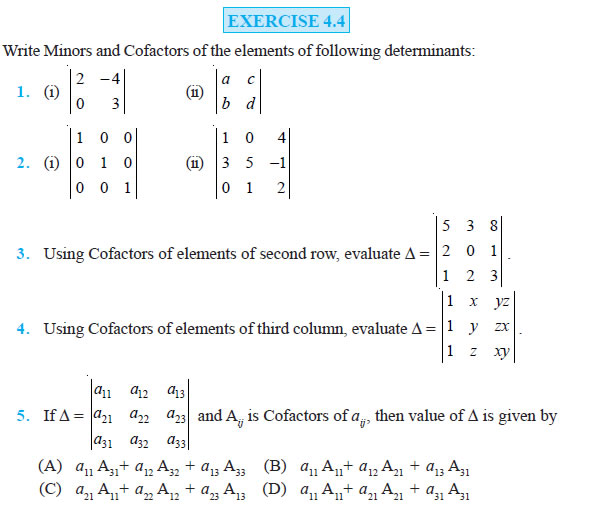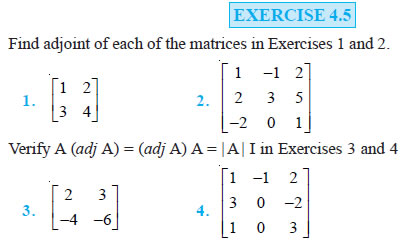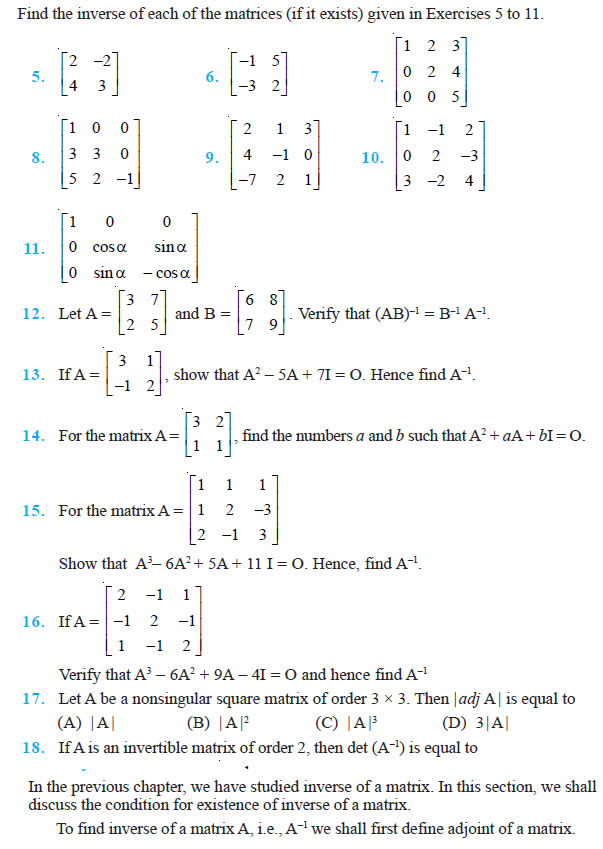### EXERCISE 4.6

Examine the consistency of the system of equations in Exercises 1 to 6.

Question 1. x + 2y = 2

Question 2. 2x – y = 5

Question 3. x + 3y = 5 2x + 3y = 3 x + y = 4 2x + 6y = 8

Question 4. x + y + z = 1

Question 5. 3x–y – 2z = 2

Question 6. 5x – y + 4z = 5 2x + 3y + 2z = 2 2y – z = –1 2x + 3y + 5z = 2 ax + ay + 2az = 4 3x – 5y = 3 5x – 2y + 6z = –1

Solve system of linear equations, using matrix method, in Exercises 7 to 14.

Question 7. 5x + 2y = 4

Question 8. 2x – y = –2

Question 9. 4x – 3y = 3 7x + 3y = 5 3x + 4y = 3 3x – 5y = 7

Question 10. 5x + 2y = 3

Question 11. 2x + y + z = 1

Question 12. x – y + z = 4 3x + 2y = 5 x – 2y – z = 3 2 2x + y – 3z = 0 3y – 5z = 9 x + y + z = 2

Question 13. 2x + 3y +3 z = 5

Question 14. x – y + 2z = 7 x – 2y + z = – 4 3x + 4y – 5z = – 5 3x – y – 2z = 3 2x – y + 3z = 12

Question 15. If A = 2 –3 5 3 2 –4 1 1 –2, find A–1. Using A–1 solve the system of equations 2x – 3y + 5z = 11 3x + 2y – 4z = – 5 x + y – 2z = – 3

Question 16. The cost of 4 kg onion, 3 kg wheat and 2 kg rice is Rs 60. The cost of 2 kg onion, 4 kg wheat and 6 kg rice is Rs 90. The cost of 6 kg onion 2 kg wheat and 3 kg rice is Rs 70. Find cost of each item per kg by matrix method.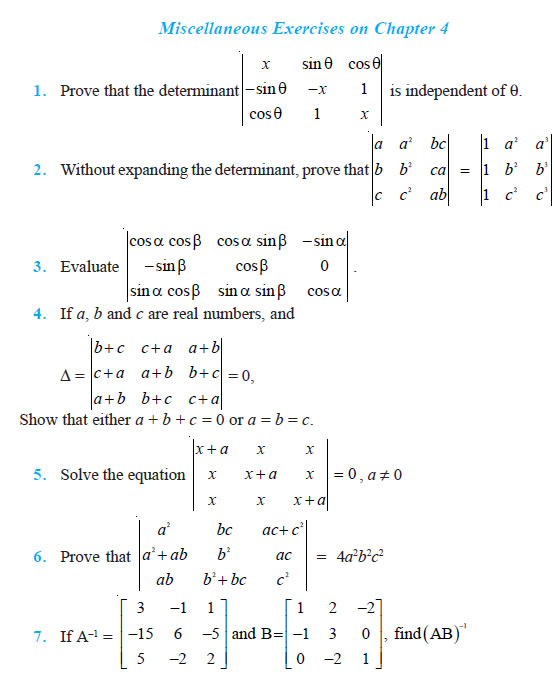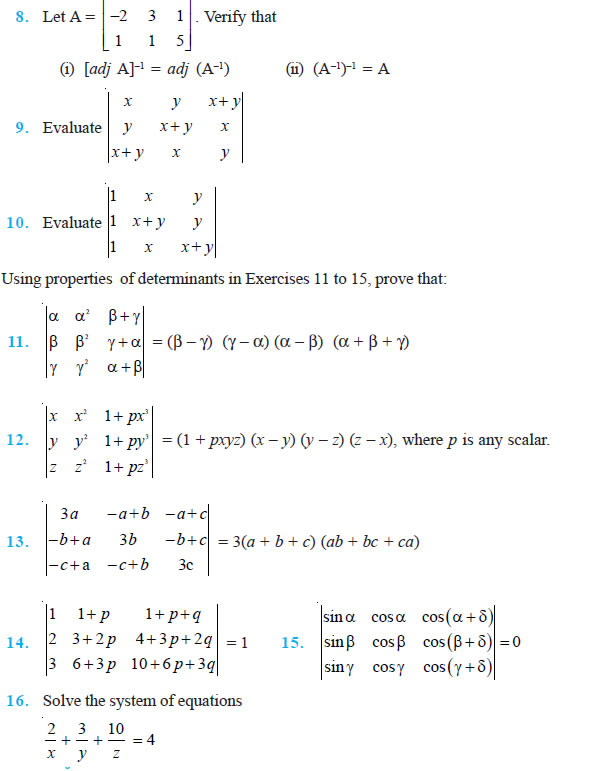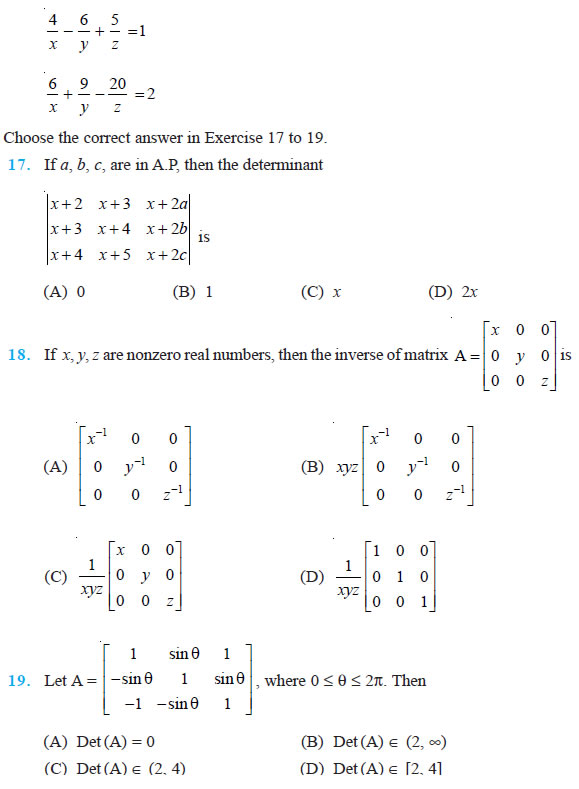### NCERT Books Free Pdf Download for Class 5, 6, 7, 8, 9, 10 , 11, 12 Hindi and English Medium

 Mathematics Biology Psychology Chemistry English Economics Sociology Hindi Business Studies Geography Science Political Science Statistics Physics Accountancy